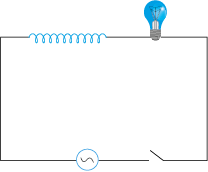Select Chapter Topics:A light bulb is rated at 100W for a 220 V AC supply. The peak voltage of the source is:

1. 220 V

2. 110 V

3. 311 V

4. 100 VSubtopic:  RMS & Average Values |
72%
To view explanation, please take trial in the course.
NEET 2023 - Target Batch - Aryan Raj Singh
Hints

A light bulb is rated at $$100$$ W for a $$220$$ V AC supply. The RMS current through the bulb is:
1. $$0.243$$ A
2. $$0.454$$ A
3. $$0.222$$ A
4. $$0.312$$ ASubtopic:  RMS & Average Values |
79%
From NCERT
To view explanation, please take trial in the course.
NEET 2023 - Target Batch - Aryan Raj Singh
Hints
To view explanation, please take trial in the course.
NEET 2023 - Target Batch - Aryan Raj Singh

A pure inductor of 25.0 mH is connected to an AC source of 220 V. The RMS current in the circuit is:

(The frequency of the source is 50 Hz).

1. 20 A

2. 25 A

3. 28 A

4. 32 ASubtopic:  RMS & Average Values |
70%
To view explanation, please take trial in the course.
NEET 2023 - Target Batch - Aryan Raj Singh
Hints

A lamp is connected in series with a capacitor. Predict your observations for dc and ac connections.

 1 When a dc source is connected to a capacitor, the lamp will not glow in a steady-state condition. 2 When an ac source is connected to a capacitor, the lamp will glow. 3 Both (1) and (2) are correct. 4 None of these.Subtopic:  Different Types of AC Circuits |
82%
To view explanation, please take trial in the course.
NEET 2023 - Target Batch - Aryan Raj Singh
Hints

A lamp is connected in series with a capacitor. AC and DC sources are connected to the circuit one by one, then mark the correct statement/s.

 1 when the DC source is connected to the capacitor, the lamp will not glow in a steady-state condition. 2 when the AC source is connected to the capacitor and the capacitance of the capacitor is reduced, the lamp will glow less brightly. 3 when the DC source is connected to the capacitor and the capacitance of the capacitor is reduced, the lamp will glow less brightly. 4 both (1) and (2).Subtopic:  Different Types of AC Circuits |
74%
From NCERT
To view explanation, please take trial in the course.
NEET 2023 - Target Batch - Aryan Raj Singh
Hints
To view explanation, please take trial in the course.
NEET 2023 - Target Batch - Aryan Raj Singh

A $$15.0~\mathrm{\mu F}$$ capacitor is connected to a $$220~\mathrm{V}$$, $$50~\mathrm{Hz}$$ source. If the frequency is doubled, what happens to the capacitive reactance and the current?

 1 the capacitive reactance is halved and the current is doubled. 2 the capacitive reactance is doubled and the current is halved. 3 the capacitive reactance remains the same and the current is doubled. 4 the current remains the same and the capacitive reactance is halved.Subtopic:  Different Types of AC Circuits |
76%
From NCERT
To view explanation, please take trial in the course.
NEET 2023 - Target Batch - Aryan Raj Singh
Hints

A light bulb and an open coil inductor are connected to an ac source through a key as shown in the figure.The switch is closed and after some time, an iron rod is inserted into the interior of the inductor. The glow of the light bulb:

1. increases

2. decreases

3. is unchanged, as the iron rod is inserted

4. first increases and then decreasesSubtopic:  Different Types of AC Circuits |
71%
To view explanation, please take trial in the course.
NEET 2023 - Target Batch - Aryan Raj Singh
Hints

A resistor of $$200~\mathrm{\Omega}$$ and a capacitor of $$15.0~\mathrm{\mu F}$$ are connected in series to a $$220~\mathrm{V}$$, $$50$$ Hz ac source. The voltage (RMS) across the resistor and the capacitor are respectively:
1. $$160.3 \mathrm{~V}, 160.3 \mathrm{~V}$$
2. $$151 \mathrm{~V}, 151 \mathrm{~V}$$
3. $$160.3 \mathrm{~V}, 151 \mathrm{~V}$$
4. $$151 \mathrm{~V}, 160.3 \mathrm{~V}$$Subtopic:  Different Types of AC Circuits |
To view explanation, please take trial in the course.
NEET 2023 - Target Batch - Aryan Raj Singh
Hints

Which one of the following is incorrect?

 1 For circuits used for transporting electric power, a low power factor implies large power loss in transmission. 2 Power factor can often be improved by the use of a capacitor of appropriate capacitance in the circuit. 3 For circuits used for transporting electric power, a low power factor implies low power loss in transmission. 4 Both (1) and (2)Subtopic:  RMS & Average Values |
To view explanation, please take trial in the course.
NEET 2023 - Target Batch - Aryan Raj Singh
Hints

A sinusoidal voltage of peak value 283 V and frequency 50 Hz is applied to a series LCR circuit in which R = 3$\Omega$L = 25.48 mH, and C = 796 µF. The impedance of the circuit is:

1. 5 $\Omega$

2. 8 $\Omega$

3. 4 $\Omega$

4. 2 $\Omega$Subtopic:  Different Types of AC Circuits |
55%
To view explanation, please take trial in the course.
NEET 2023 - Target Batch - Aryan Raj Singh
Hints